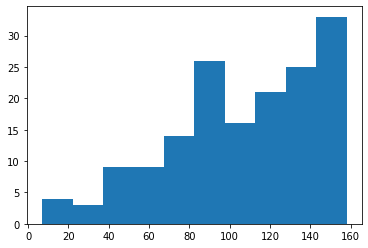Callbacks which work with a learner's data
from nbdev.showdoc import *
from fastai2.test_utils import *


## classCollectDataCallback[source]

CollectDataCallback(begin_fit=None, begin_epoch=None, begin_train=None, begin_batch=None, after_pred=None, after_loss=None, after_backward=None, after_step=None, after_cancel_batch=None, after_batch=None, after_cancel_train=None, after_train=None, begin_validate=None, after_cancel_validate=None, after_validate=None, after_cancel_epoch=None, after_epoch=None, after_cancel_fit=None, after_fit=None) :: Callback

Collect all batches, along with pred and loss, into self.data. Mainly for testing

## classCudaCallback[source]

CudaCallback(device=None) :: Callback

Move data to CUDA device

You don't normally need to use this Callback, because fastai's DataLoader will handle passing data to a device for you. However, if you already have a plain PyTorch DataLoader and can't change it for some reason, you can use this transform.

learn = synth_learner(cbs=CudaCallback)
learn.model
learn.fit(1)
test_eq(next(learn.model.parameters()).device.type, 'cuda')

(#4) [0,12.672515869140625,9.3746976852417,'00:00']


## classWeightedDL[source]

WeightedDL(dataset=None, bs=None, wgts=None, shuffle=False, num_workers=None, verbose=False, do_setup=True, pin_memory=False, timeout=0, batch_size=None, drop_last=False, indexed=None, n=None, device=None, wif=None, before_iter=None, after_item=None, before_batch=None, after_batch=None, after_iter=None, create_batches=None, create_item=None, create_batch=None, retain=None, get_idxs=None, sample=None, shuffle_fn=None, do_batch=None) :: TfmdDL

Transformed DataLoader

#### Datasets.weighted_dataloaders[source]

Datasets.weighted_dataloaders(wgts, bs=64, val_bs=None, shuffle_train=True, n=None, path='.', dl_type=None, dl_kwargs=None, device=None)

n = 160
dsets = Datasets(torch.arange(n).float())
learn = synth_learner(data=dls, cbs=CollectDataCallback)

learn.fit(1)
t = concat(*learn.collect_data.data.itemgot(0,0))
plt.hist(t);

(#4) [0,nan,None,'00:00']## classPartialDL[source]

PartialDL(dataset=None, bs=None, partial_n=None, shuffle=False, num_workers=None, verbose=False, do_setup=True, pin_memory=False, timeout=0, batch_size=None, drop_last=False, indexed=None, n=None, device=None, wif=None, before_iter=None, after_item=None, before_batch=None, after_batch=None, after_iter=None, create_batches=None, create_item=None, create_batch=None, retain=None, get_idxs=None, sample=None, shuffle_fn=None, do_batch=None) :: TfmdDL

Select randomly partial quantity of data at each epoch

#### FilteredBase.partial_dataloaders[source]

FilteredBase.partial_dataloaders(partial_n, bs=64, val_bs=None, shuffle_train=True, n=None, path='.', dl_type=None, dl_kwargs=None, device=None)

Create a partial dataloader PartialDL for the training set

dls = dsets.partial_dataloaders(partial_n=32, bs=16)

assert len(dls)==2
for batch in dls:
assert len(batch)==16